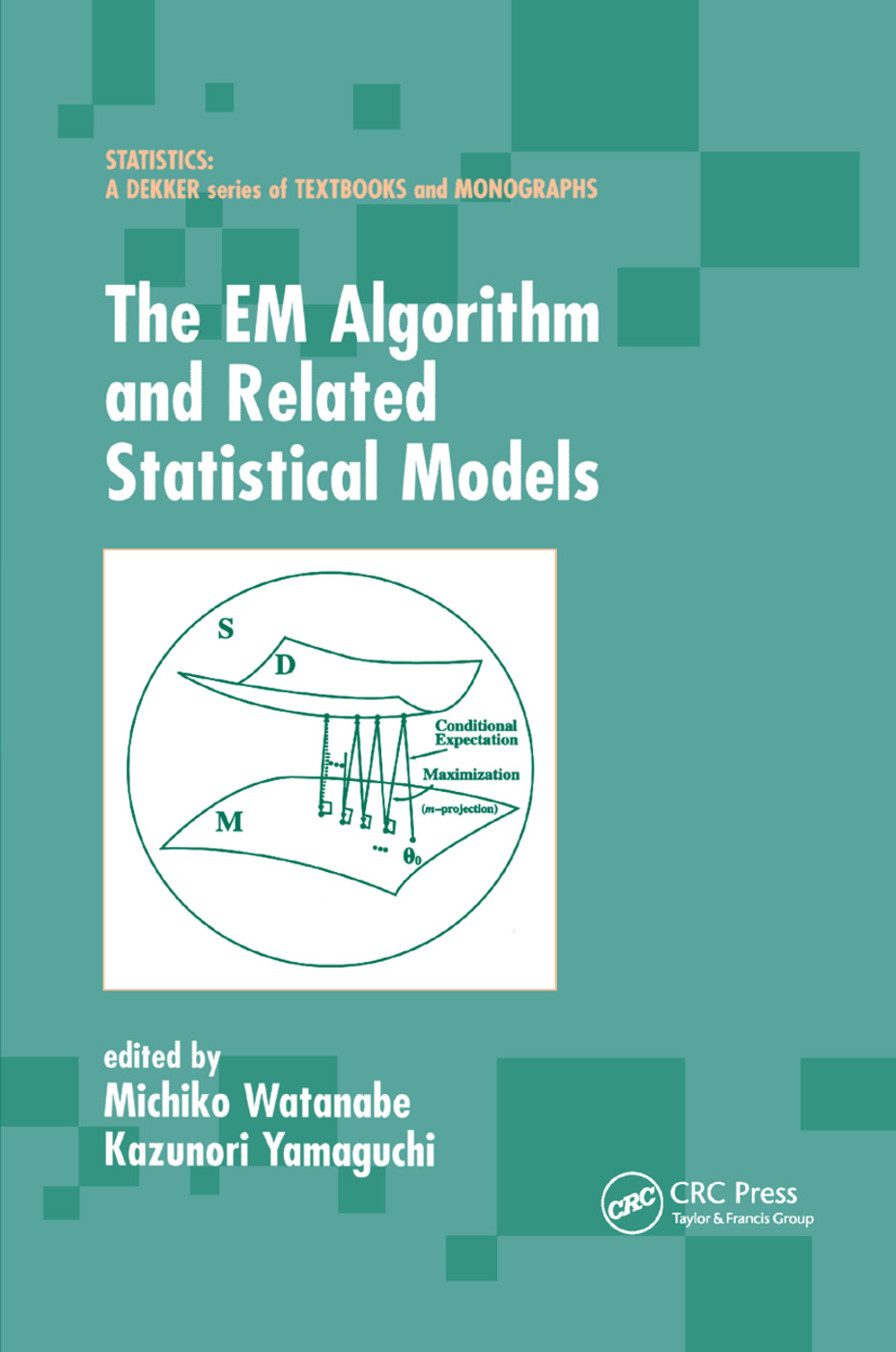# The EM Algorithm and Related Statistical Models

## 1st Edition

CRC Press

250 pages

Paperback: 9780367394936
pub: 2019-10-17
SAVE ~\$14.99
\$74.95
\$59.96
x
Hardback: 9780824747015
pub: 2003-10-15
SAVE ~\$29.00
\$145.00
\$116.00
x
eBook (VitalSource) : 9780429223617
pub: 2003-10-15
from \$37.48

FREE Standard Shipping!

### Description

Exploring the application and formulation of the EM algorithm, The EM Algorithm and Related Statistical Models offers a valuable method for constructing statistical models when only incomplete information is available, and proposes specific estimation algorithms for solutions to incomplete data problems. The text covers current topics including statistical models with latent variables, as well as neural network models, and Markov Chain Monte Carlo methods. It describes software resources valuable for the processing of the EM algorithm with incomplete data and for general analysis of latent structure models of categorical data, and studies accelerated versions of the EM algorithm.

Incomplete data and the generation mechanisms; type of incomplete data and its analysis; statistical models for incomplete data; analysis of data with missing values; missing data in multinominal data; algorithms for MLE for multivariate normal datawith missing values; scoring method; EM algorithm; basics of EM algorithm; extension of EM algorithm and acceleration; EM algorithm as an optimization tool robust model and outlier detection; scale mixture model of normal distributions; multivariate andcontaminated normal distribution; robust tobit model; robust factor model; statistical model with latent variables; latent structure model and EM algorithm; latent class model and latent trait model; structured equations model with latent variables;extensions of EM algorithm; ECM algorithm; ECME algorithm; optimal EM algorithm; MCEM algorithm; covergence speed of EM algorithm; convergence speed; comparisons of EM and other optimization algorithms quasi Newton method; acceleration methods of the EMalgorithm; neural networks and EM algorithm; EM algorithm in neural networks; geometric interpretation of EM algorithm; Marcov chain Monte Carlo; Bayes estimation; Marcov chain; Metropolis-Hastings algorithm; data augmentation algorithm; poor man's dataaugmentation algorithm; Gibbs sampling algorithm. Appendices: SOLAS for missing data analysis; Lem.

Michiko Watanabe, Kazunori Yamaguchi